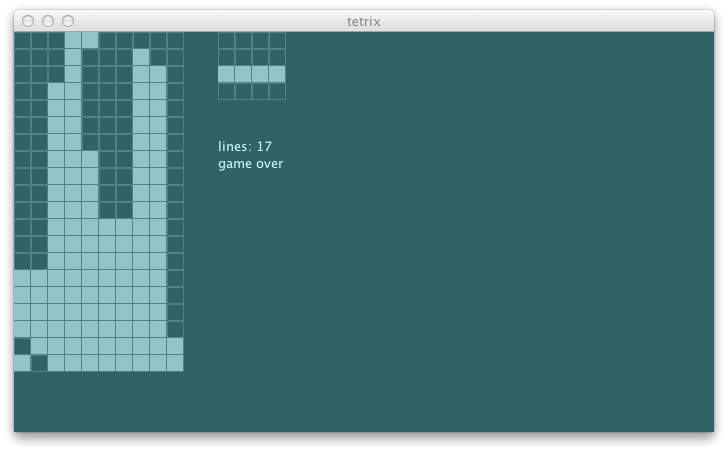### cavity

One thing I’ve been noticing as the agent plays the game is that it is happy to make cavities. A cavity is created when one or more block exists above an unfilled spot.

``````-fig 1----

xxxx
xxxx x xxx
x x xxxxxx
----------
``````

Likely to avoid the height penalty, it would for example lay out the I bar flat on top of uneven surface instead of dropping it upright. To minimize the cavity, I’d like it to play:

``````-fig 2-----

x
x  xxxx
x x xxx
x xxxxxxxx
----------
``````

Let’s cauculate the height penalties from the first four columns.

``````scala> val fig1 = math.sqrt(List(2, 2, 2, 2) map { x => x * x } sum)
fig1: Double = 4.0

scala> val fig2 = math.sqrt(List(1, 0, 4, 1) map { x => x * x } sum)
fig2: Double = 4.242640687119285
``````

As predicted, fig1 will incur lower penalty. We can create additional penalty for all blocks covering another block using its height * height. Then the new penalty becomes as follows:

``````scala> val fig1b = math.sqrt(List(2, 2, 2, 2, 2, 2) map { x => x * x } sum)
fig1b: Double = 4.898979485566356

scala> val fig2b = math.sqrt(List(1, 0, 4, 1) map { x => x * x } sum)
fig2b: Double = 4.242640687119285
``````

This time, fig2 is preferred. Let’s write this in spec:

``````                                                                              s2"""
Penalty function should
penalize having blocks stacked up high                                    \$penalty1
penalize having blocks covering other blocks                              \$penalty2
"""
...
def penalty2 = {
val s = newState(Seq(
(0, 0), (2, 0), (0, 1), (1, 1), (2, 1), (3, 1))
map { Block(_, TKind) }, (10, 20), TKind :: TKind :: Nil)
agent.penalty(s) must beCloseTo(4.89, 0.01)
}
``````

The test fails as expected:

``````[info] Penalty function should
[info] + penalize having blocks stacked up high
[error] x penalize having blocks covering other blocks
[error]    4.0 is not close to 4.89 +/- 0.01 (AgentSpec.scala:13)
``````

Let’s implement this using the REPL:

``````scala>     val s = newState(Seq(
(0, 0), (2, 0), (0, 1), (1, 1), (2, 1), (3, 1))
map { Block(_, TKind) }, (10, 20), TKind :: TKind :: Nil)
s: com.eed3si9n.tetrix.GameState = GameState(List(Block((0,0),TKind), Block((2,0),TKind),
Block((0,1),TKind), Block((1,1),TKind), Block((2,1),TKind), Block((3,1),TKind),
Block((4,18),TKind), Block((5,18),TKind), Block((6,18),TKind), Block((5,19),TKind)),(10,20),
Piece((5.0,18.0),TKind,List((-1.0,0.0), (0.0,0.0), (1.0,0.0), (0.0,1.0))),
Piece((2.0,1.0),TKind,List((-1.0,0.0), (0.0,0.0), (1.0,0.0), (0.0,1.0))),List(),ActiveStatus,0)

scala> val groupedByX = s.unload(s.currentPiece).blocks map {_.pos} groupBy {_._1}
groupedByX: scala.collection.immutable.Map[Int,Seq[(Int, Int)]] = Map(
3 -> List((3,1)), 1 -> List((1,1)), 2 -> List((2,0), (2,1)), 0 -> List((0,0), (0,1)))

scala> groupedByX map { case (k, vs) => vs.map(_._2).sorted.zipWithIndex }
res14: scala.collection.immutable.Iterable[Seq[(Int, Int)]] = List(
List((1,0)), List((1,0)), List((0,0), (1,1)), List((0,0), (1,1)))

scala> groupedByX map { case (k, vs) =>
vs.map(_._2).sorted.zipWithIndex.dropWhile(x => x._1 == x._2) }
res15: scala.collection.immutable.Iterable[Seq[(Int, Int)]] = List(
List((1,0)), List((1,0)), List(), List())

scala> val coverups = groupedByX flatMap { case (k, vs) =>
vs.map(_._2).sorted.zipWithIndex.dropWhile(x => x._1 == x._2).map(_._1 + 1) }
coverups: scala.collection.immutable.Iterable[Int] = List(2, 2)
``````

Here’s the resulting `penalty`:

``````  def penalty(s: GameState): Double = {
val groupedByX = s.unload(s.currentPiece).blocks map {_.pos} groupBy {_._1}
val heights = groupedByX map { case (k, v) => v.map({_._2 + 1}).max }
val coverups = groupedByX flatMap { case (k, vs) =>
vs.map(_._2).sorted.zipWithIndex.dropWhile(x => x._1 == x._2).map(_._1 + 1) }
math.sqrt( (heights ++ coverups) map { x => x * x } sum)
}
``````

This passes the test:

``````[info]   Penalty function should
[info]     + penalize having blocks stacked up high
[info]     + penalize having blocks covering other blocks
``````

Let’s see how it plays:The cavity avoidance is working, but almost too well. Maybe we should reinstate the gap penalty tomorrow.

``````\$ git fetch origin
\$ git co day9v2 -b try/day9
\$ sbt swing/run
``````